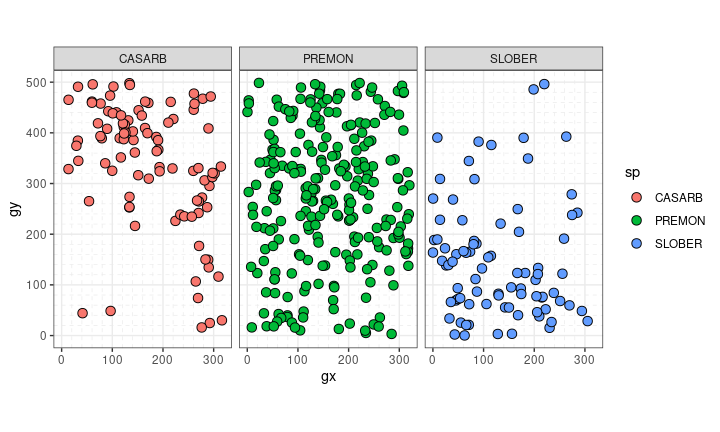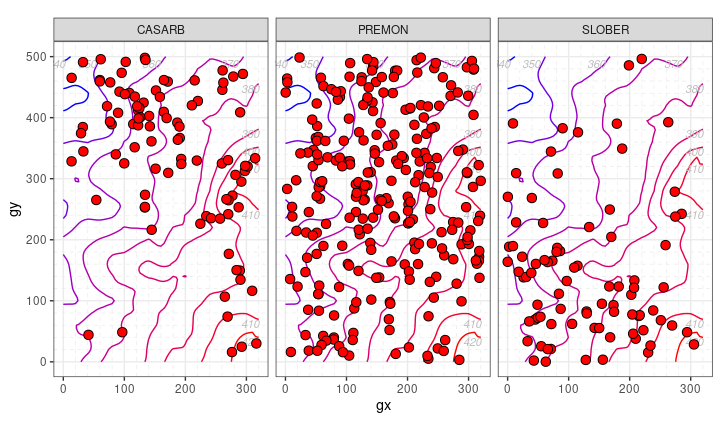#Plot ForestGEO data

fgeo.plot helps you to plot ForestGEO data.

## Installation

Install the latest stable version of fgeo.plot from CRAN with:

``install.packages("fgeo.plot")``

Or install the development version of fgeo.plot with:

``````# install.packages("devtools")
devtools::install_github("forestgeo/fgeo.plot")``````

## Example

``````library(fgeo.plot)

small_census <- fgeo.x::tree6_3species

autoplot(sp(small_census))````````````elevation <- fgeo.x::elevation

autoplot(
sp_elev(small_census, elevation),
fill = "red",
hide_color_legend = TRUE
)``````Get started with fgeo